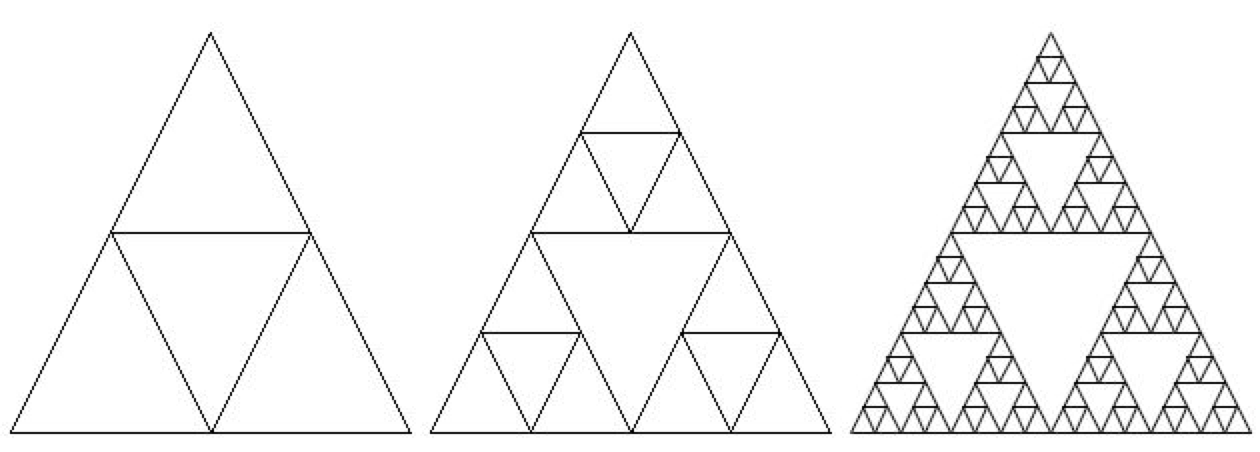# 12.3. Why use Recursion?¶

Recursion is most useful when it is used to solve problems where the structure of the problem repeats. For example, what if you wanted to find out how much space a folder on your computers uses? You could add up the sizes of all the files in that folder, but folders can also contain subfolders. So you will have to repeat the procedure (method) for each subfolder. Each subfolder can also contain subfolders.

Recursion can also be used to create fractals. A simple example is Sierpinski’s triangle in which you subdivide a triangle into 4 new triangles as shown below. You can then do the some procedure with each new triangle except the center one.Figure 1: A sequence of Sierpinski’s triangles